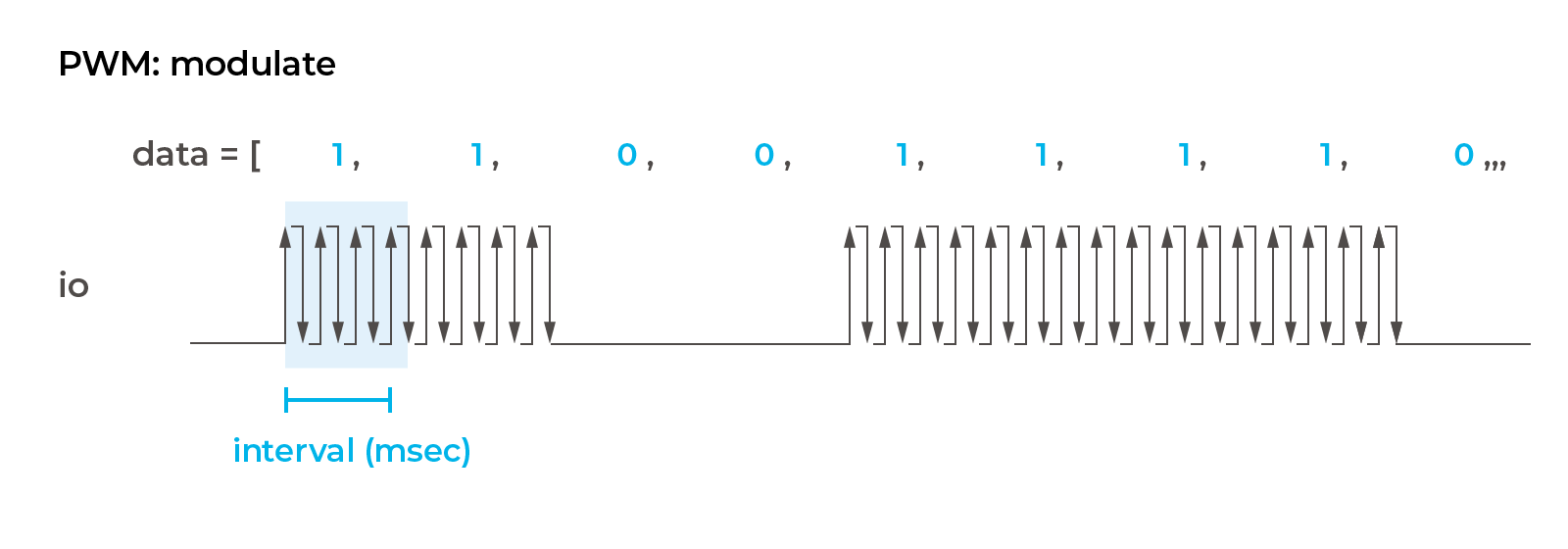# Modulation

Modulation is a function to modulate using PWM. obniz implements AM modulation. This is most often used with obniz for infrared remote control signal transmission. As for the infrared remote control, the signal is sent by digitally modulating the 38KHz frequency with the data you want to send.

## AM modulation

As shown in the figure below, when the data to be sent is 1, the PWM is output, and when it is 0, nothing is output; the output is false.Modulation starts by calling pwm.modulate() on the working PWM. The IO and the frequency are fixed, but the duty ratio is 50% output no matter what value is set. pwm.modulate() sets "am" and then sets the time (msec) for 1 bit. In the example below, it is 1 msec, so it takes 8 msec to send 8 bits. In the end, the data you want to send is written as an array, which is separated by every bits.

``````// Javascript Example

var pwm = obniz.getFreePwm();
pwm.start({io:0});
pwm.freq(5000);
pwm.modulate("am", 1, [1,1,0,0,1,1,1,0]);
``````